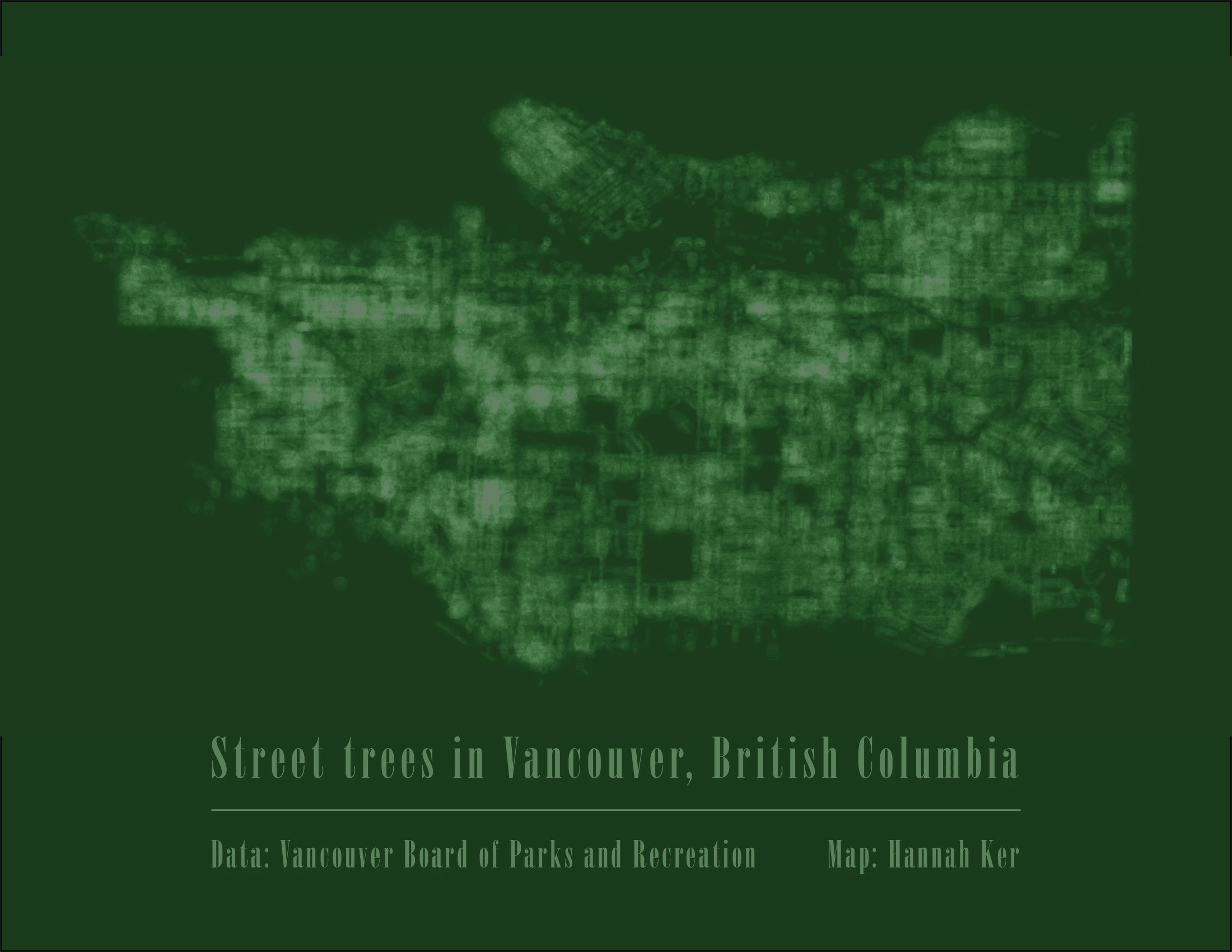# A minimal map of Vancouver’s street treesI have been a long-time admirer of the ‘Minimal Maps’ series from Michael Pecirno, and wanted to try to recreate something similar. I really liked his focus on the natural environment and spent a while exploring potential datasets, at a variety of scales. I eventually settled on this dataset of street trees in Vancouver. This dataset has one point for every public tree on Vancouver’s boulevards (there are over 140,000!).

### Data Cleaning

I downloaded this dataset as a CSV (it was taking too long to open in R as a GeoJSON) and read it into R.

``````# loading in necessary libraries
library(ggplot2)
library(rlist)
library(sf)

# open the dataset
trees <- read.csv('street-trees.csv', sep = ';')``````

Because I am still finding R’s factor data type challenging to work with, I converted everything into integers or strings. I also removed the entries without any coordinates.

``````# convert factors to strings
i <- sapply(trees, is.factor) # find columns that are factors
trees[i] <- lapply(trees[i], as.character) # convert column to character

# remove rows without coordinates
new <- subset(trees, nchar(Geom) > 1)``````

The most challenging part of this process was getting the coordinate data into a workable format. Initially, this data for each tree was stored in a cell in a format like this:

{\“type\”: \“Point\“, \“coordinates\”: [-123.135032, 49.230858]}

I wrote a function to parse these strings, one at a time, and return a list that contained just the coordinates.

``````# function to parse strings to get coordinates
getcoords <- function(string){

split <- strsplit(string,']')[]
a <- split[]
b <- strsplit(a, '-')[][]
c <- strsplit(b, ',')
d <- c[]

x <- paste0('-', d[])
y <- strsplit(d[], ' ')[][]

coords <- list(x,y)
return(coords)

}``````

I applied this function to each point in the dataset and created new columns for each coordinate.

``````# apply the function for each row
for (i in 1:length(new\$Geom)){

# parse to get the coordinates
z <- getcoords(new\$Geom[[i]])
x <- z[]
y <- z[]

# add new columns to df
new\$lats[i] <- x
new\$longs[i] <- y

}

# convert the columns from characters to nums
new\$lats <- as.numeric(new\$lats)
new\$longs<- as.numeric(new\$longs)``````

### Visualizing the data

Once all the data was cleaned, it was a very simple matter to visualize using ggplot2. Given that the dataset was decently large, I took a smaller subset of all points for faster visualization while I tested different visualization parameters.

``````# get smaller version for faster testing
small <- new[1:2500,]

# make the map
map <- ggplot(data=small) +
geom_point(data=small,
aes(x=small\$lats,
y=small\$longs),
size=small\$DIAMETER*0.1,
alpha=0.005,
color='#ffffff')+
theme_map()``````

After outputting this map, I used inDesign to add some simple text for a title.